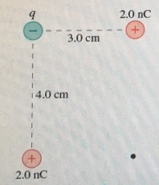# Problem: What is the electric potential at the point indicated with the dot in the figure below? Suppose that q = 1.4 nC.

###### FREE Expert Solution

Electric potential due to charge:

$\overline{){\mathbf{V}}{\mathbf{=}}\frac{\mathbf{k}\mathbf{q}}{\mathbf{r}}}$

The potential at the point will be addition of the potentials due to all the three charges.

The distance between the charge q and the point is given by:

${\mathbit{r}}_{\mathbf{q}\mathbf{p}}\mathbf{=}\sqrt{\mathbf{3}\mathbf{.}{\mathbf{0}}^{\mathbf{2}}\mathbf{+}\mathbf{4}\mathbf{.}{\mathbf{0}}^{\mathbf{2}}}$

rqp = 5cm.

85% (366 ratings)###### Problem Details

What is the electric potential at the point indicated with the dot in the figure below? Suppose that q = 1.4 nC.Frequently Asked Questions

What scientific concept do you need to know in order to solve this problem?

Our tutors have indicated that to solve this problem you will need to apply the Electric Potential concept. You can view video lessons to learn Electric Potential. Or if you need more Electric Potential practice, you can also practice Electric Potential practice problems.

What professor is this problem relevant for?

Based on our data, we think this problem is relevant for Professor Bauer's class at UCLA.